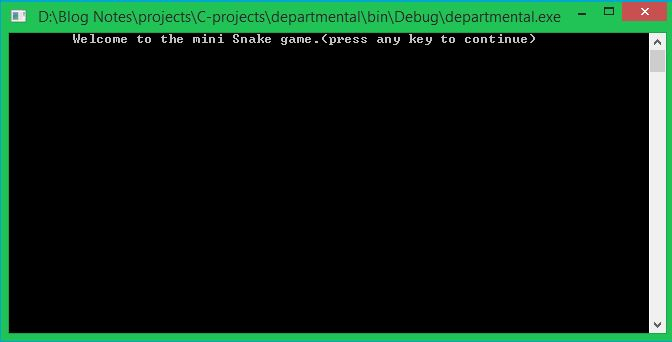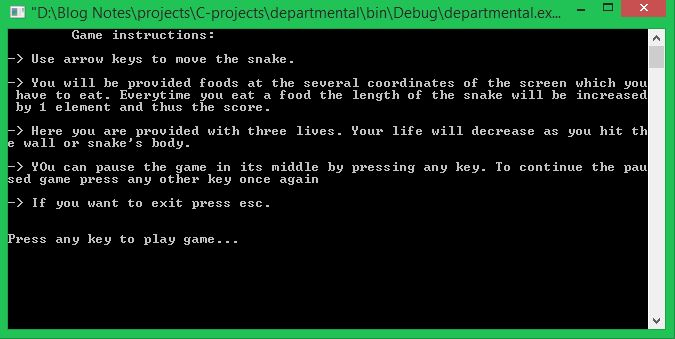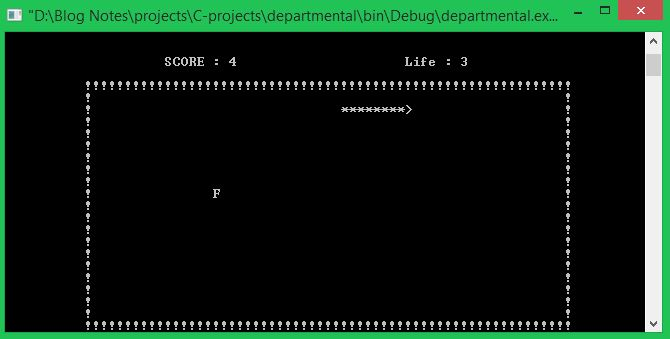# C Projects Source Code - Snake Game

Published October 22, 2021

In this c projects source code series we are going to create a simple Snake game with C Language. It is also a c console-based application, which is designed simply without using graphics. Snake Game Project is built for entertainment purposes like the Pacman game. The user gets a realistic feeling of playing the mobile snake game through this application.

The various coordinates in the screen have the food for the snake to eat, and when the snake eats food, it gains length by one element. The player gets their score increased according to the food that the snake consumes.

Three lives are given for the player, and when it is over the game will be terminated automatically

Snake Game imagesThe complete code for Snake Game with c Language

 ```//-PROGRAMMING MADE SIMPLE #include #include #include #include #include #include #include #include #include #define UP 72 #define DOWN 80 #define LEFT 75 #define RIGHT 77 int length; int bend_no; int len; char key; void record(); void load(); int life; void Delay(long double); void Move(); void Food(); int Score(); void Print(); void gotoxy(int x, int y); void GotoXY(int x,int y); void Bend(); void Boarder(); void Down(); void Left(); void Up(); void Right(); void ExitGame(); int Scoreonly(); struct coordinate { int x; int y; int direction; }; typedef struct coordinate coordinate; coordinate head, bend,food,body; int main() { char key; Print(); system("cls"); load(); length=5; head.x=25; head.y=20; head.direction=RIGHT; Boarder(); Food(); //to generate food coordinates initially life=3; //number of extra lives bend=head; Move(); //initialing initial bend coordinate return 0; } void Move() { int a,i; do { Food(); fflush(stdin); len=0; for(i=0; i<30; i++) { body[i].x=0; body[i].y=0; if(i==length) break; } Delay(length); Boarder(); if(head.direction==RIGHT) Right(); else if(head.direction==LEFT) Left(); else if(head.direction==DOWN) Down(); else if(head.direction==UP) Up(); ExitGame(); } while(!kbhit()); a=getch(); if(a==27) { system("cls"); exit(0); } key=getch(); if((key==RIGHT&&head.direction!=LEFT&&head.direction!=RIGHT)||(key==LEFT&&head.direction!=RIGHT&&head.direction!=LEFT)||(key==UP&&head.direction!=DOWN&&head.direction!=UP)||(key==DOWN&&head.direction!=UP&&head.direction!=DOWN)) { bend_no++; bend[bend_no]=head; head.direction=key; if(key==UP) head.y--; if(key==DOWN) head.y++; if(key==RIGHT) head.x++; if(key==LEFT) head.x--; Move(); } else if(key==27) { system("cls"); exit(0); } else { printf("\a"); Move(); } } void gotoxy(int x, int y) { COORD coord; coord.X = x; coord.Y = y; SetConsoleCursorPosition(GetStdHandle(STD_OUTPUT_HANDLE), coord); } void GotoXY(int x, int y) { HANDLE a; COORD b; fflush(stdout); b.X = x; b.Y = y; a = GetStdHandle(STD_OUTPUT_HANDLE); SetConsoleCursorPosition(a,b); } void load() { int row,col,r,c,q; gotoxy(36,14); printf("loading..."); gotoxy(30,15); for(r=1; r<=20; r++) { for(q=0; q<=100000000; q++); //to display the character slowly printf("%c",177); } getch(); } void Down() { int i; for(i=0; i<=(head.y-bend[bend_no].y)&&len=70||head.y<=10||head.y>=30||check!=0) { life--; if(life>=0) { head.x=25; head.y=20; bend_no=0; head.direction=RIGHT; Move(); } else { system("cls"); printf("All lives completed\nBetter Luck Next Time!!!\nPress any key to quit the game\n"); record(); exit(0); } } } void Food() { if(head.x==food.x&&head.y==food.y) { length++; time_t a; a=time(0); srand(a); food.x=rand()%70; if(food.x<=10) food.x+=11; food.y=rand()%30; if(food.y<=10) food.y+=11; } else if(food.x==0)/*to create food for the first time coz global variable are initialized with 0*/ { food.x=rand()%70; if(food.x<=10) food.x+=11; food.y=rand()%30; if(food.y<=10) food.y+=11; } } void Left() { int i; for(i=0; i<=(bend[bend_no].x-head.x)&&len"); else printf("*"); } /*body[len].x=head.x-i; body[len].y=head.y;*/ len++; } Bend(); if(!kbhit()) head.x++; } void Bend() { int i,j,diff; for(i=bend_no; i>=0&&len0) for(j=1; j<=diff; j++) { /*GotoXY(bend[i].x,(bend[i].y-j)); printf("*");*/ body[len].x=bend[i].x; body[len].y=bend[i].y-j; GotoXY(body[len].x,body[len].y); printf("*"); len++; if(len==length) break; } } else if(bend[i].y==bend[i-1].y) { diff=bend[i].x-bend[i-1].x; if(diff<0) for(j=1; j<=(-diff)&&len0) for(j=1; j<=diff&&len Use arrow keys to move the snake.\n\n-> You will be provided foods at the several coordinates of the screen which you have to eat. Everytime you eat a food the length of the snake will be increased by 1 element and thus the score.\n\n-> Here you are provided with three lives. Your life will decrease as you hit the wall or snake's body.\n\n-> YOu can pause the game in its middle by pressing any key. To continue the paused game press any other key once again\n\n-> If you want to exit press esc. \n"); printf("\n\nPress any key to play game..."); if(getch()==27) exit(0); } void record() { char plname,nplname,cha,c; int i,j,px; FILE *info; info=fopen("record.txt","a+"); getch(); system("cls"); printf("Enter your name\n"); scanf("%[^\n]",plname); //************************ for(j=0; plname[j]!='\0'; j++) //to convert the first letter after space to capital { nplname=toupper(plname); if(plname[j-1]==' ') { nplname[j]=toupper(plname[j]); nplname[j-1]=plname[j-1]; } else nplname[j]=plname[j]; } nplname[j]='\0'; //***************************** //sdfprintf(info,"\t\t\tPlayers List\n"); fprintf(info,"Player Name :%s\n",nplname); //for date and time time_t mytime; mytime = time(NULL); fprintf(info,"Played Date:%s",ctime(&mytime)); //************************** fprintf(info,"Score:%d\n",px=Scoreonly());//call score to display score //fprintf(info,"\nLevel:%d\n",10);//call level to display level for(i=0; i<=50; i++) fprintf(info,"%c",'_'); fprintf(info,"\n"); fclose(info); printf("Wanna see past records press 'y'\n"); cha=getch(); system("cls"); if(cha=='y') { info=fopen("record.txt","r"); do { putchar(c=getc(info)); } while(c!=EOF); } fclose(info); } int Score() { int score; GotoXY(20,8); score=length-5; printf("SCORE : %d",(length-5)); score=length-5; GotoXY(50,8); printf("Life : %d",life); return score; } int Scoreonly() { int score=Score(); system("cls"); return score; } void Up() { int i; for(i=0; i<=(bend[bend_no].y-head.y)&&len

Conclusion: This Snake Game is a c console-based application, which is designed simply without using graphics. Snake Game Project is built for entertainment purposes like the Pacman game. The user gets a realistic feeling of playing the mobile snake game through this application

 Article Contributed By :1914 Views
Projects By category
Categories